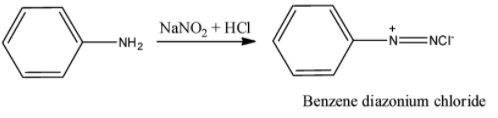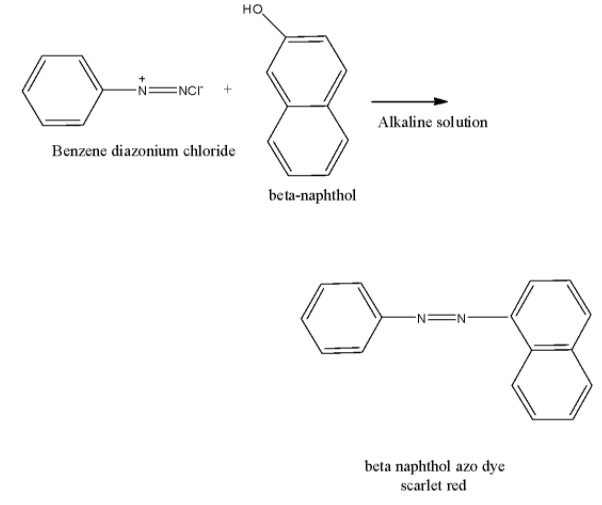QuestionAnswers

# For the identification of beta $\beta$-naphthol using dye test, it is necessary to use:A. dichloromethane solution of $\beta$-naphtholB. acidic solution of $\beta$-naphtholC. neutral solution of$\beta$-naphtholD. alkaline solution of $\beta$-naphthol

Hint: We know that azo dye test is used for identification of aromatic primary amines. Primary amine is the amine which is in the form of ${\rm{R}} - {\rm{N}}{{\rm{H}}_{\rm{2}}}$ (R is any alkyl group).

The aromatic primary amine can be confirmed by azo dye test. In this test, primary aromatic amine like aniline reacts with nitrous acid (produced by reaction of sodium nitrite with hydrochloric acid) at zero to five degree Celsius to form diazonium salt or benzene diazonium chloride. The reaction of formed benzene diazonium chloride with $\beta$-naphthol gives a scarlet red dye and this die is sparingly soluble in water. The reaction can be shown as follows:Let’s come to the question. We have to identify the type of solution of $\beta$-naphthol to be used in dye test. If the solution of $\beta$-naphthol is alkaline, -OH convert to ${{\rm{O}}^ - }$ which reacts with azo compound (benzene diazonium chloride) to form azo dye. The chemical reaction is shown below.Hence, the correct option is D, that is, an alkaline solution.

Additional Information Another test of amine is carbylamine test. In this test aromatic as well as aliphatic primary amine is heated with chloroform to result in carbylamine. The reaction is,

${\rm{R}} - {\rm{N}}{{\rm{H}}_{\rm{2}}} + {\rm{CHC}}{{\rm{l}}_{\rm{3}}} + 3{\rm{KOH}} \to {\rm{RNC}}\left( {{\rm{Carbylamine}}} \right) + 3{\rm{KCl + 3}}{{\rm{H}}_2}{\rm{O}}$

Note: It is to be noted that aromatic primary can be distinguished from secondary and tertiary amine by azo dye test and aliphatic or aromatic primary amine can be differentiated from secondary and tertiary amine by Carbylamine test.